## Example Questions

1 2 4 Next →

### Example Question #31 : How To Multiply Exponents

Simplify: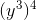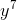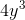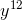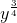Explanation:

When an exponent is raised to an exponent, multiply the two together to yield a new exponent, and attach that exponent to the original base.

In this case, multiplyandto yield, and your answer is.

### Example Question #32 : How To Multiply Exponents

Solve: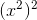when.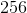Explanation:

When an exponent is raised to another exponent, you may multiply them together to find the exponent of the answer. Attach that exponent to the original base, and that is the solution.

In this particular problem, multiplyby, which yieldsas the final exponent. This makes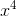.

Now, substitutefor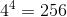.

### Example Question #33 : How To Multiply Exponents

What is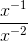?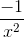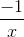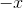Explanation:

Negative exponents are reciprocals. Do not change the sign to negative.

The variables with negative exponents can be written as their reciprocals.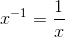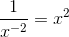,

Multiplying these new forms together gives,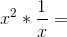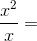From here, simplify by factoring out a common factor from the numerator and denominator.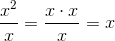### Example Question #34 : How To Multiply Exponents

Simplify the following: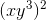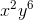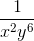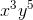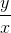Explanation:

To solve, simply distribute the outer exponent. When you have an exponent to an exponent, you multiply their values. Thus,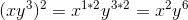### Example Question #35 : How To Multiply Exponents

Which of the following is a value of x that satisfies4

1

0

3

2

2

Explanation:

This question incorporates properties of exponents. The best way to solve this problem is to establish the same base for all of the terms.Now use the properties of exponents to simplify the left side of the equation. When exponential terms with the same base are multiplied, the exponents are added.Now, with the left side simplified, set that equal to.Since each side has one term with the same base, simply set the exponents equal to each other and solve for x.1 2 4 Next →